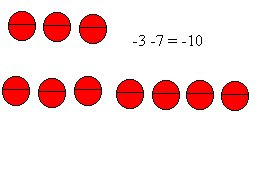## Thursday, March 17, 2011

### Jocelyn's great book of Integers

Chapter 1 :-3-(-7)=4

-3-7=-10Chapter 2:

Multiplying Integers

(+2) x (+3)= 6
(+2) x (-3)= -6

(-2) x (+3)= -6

(-2) x (-3)= 6

(+2) x (+3) = 6

(+2) x (-3) = -6

(-2) x (+3) = -6

-2) x (-3) = 6

ODD- If there are an odd amount of negative numbers(-) the product will be NEGATIVE

EVEN- If there are an even amount of negative numbers(-) the product will be POSITIVE

Chapter 3:

The same rule applies for Dividing integers

Dividing Integers

(+2) x (+3)= 6

(+2) x (-3)= -6

(-2) x (+3)= -6

(-2) x(-3)= 6

(+2) x (+3)= 6

(+2) x (-3)= -6

(-2) x (+3)= -6

(-2) x (-3)= 6

Chapter 4:
Order of Operations with Integers

Steps to solve (+5) x (-3) + (-6) ÷ (+3)=
1. Put square brackets around the operation that has to be done first (multiplication, division) 2. Solve the problem in the brackets and add the rest of the problem using the new number from the"brackets" 3. Next step is the same if there are anymore multiplication or division signs left 4.If there are no more multiplaction or division signs then you could finish by solving the rest of the problem using the addition and subtraction signs